• Slides: 33Watch the Youtube video below: https: //www. youtube. com/wat ch? v=6 mop. Agqjk. VM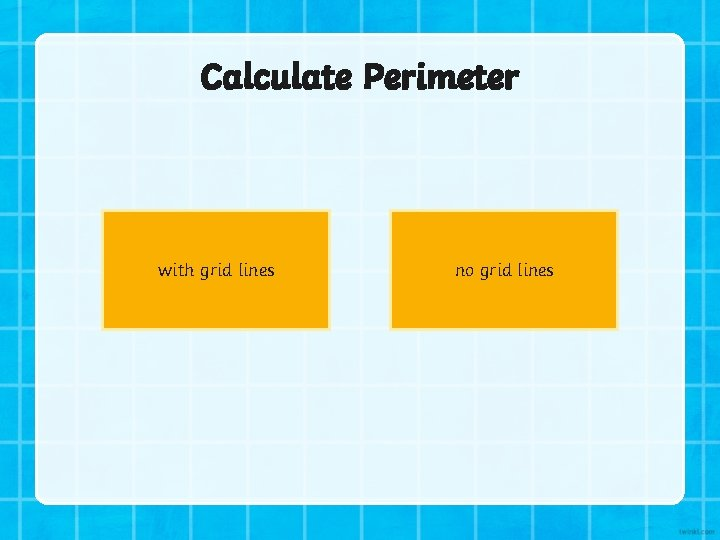Calculate Perimeter with grid lines no grid lines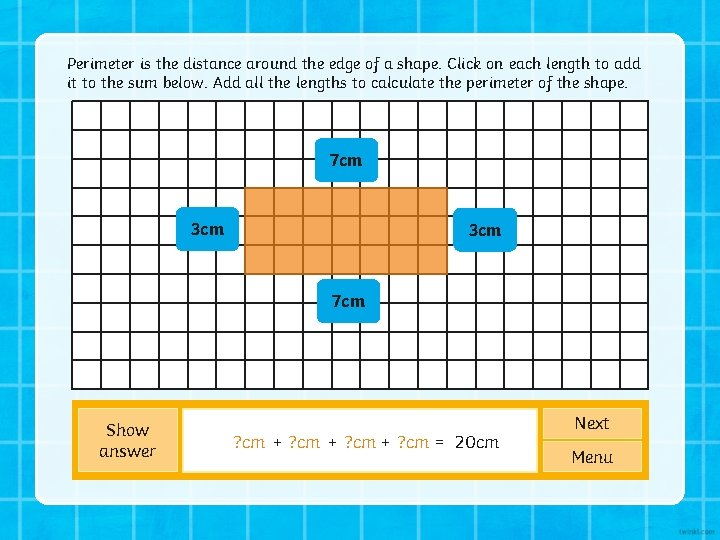Perimeter is the distance around the edge of a shape. Click on each length to add it to the sum below. Add all the lengths to calculate the perimeter of the shape. 7 cm 3 cm 7 cm Show answer ? cm + 3 cm ? cm = 20 cm 7 cm + ? cm 3 cm + 7 cm Next MenuPerimeter is the distance around the edge of a shape. Click on each length to add it to the sum below. Add all the lengths to calculate the perimeter of the shape. 6 cm 5 cm 6 cm Show answer ? cm + 5 cm ? cm + 6 cm ? cm + 5 cm ? cm = 22 cm 6 cm Next Menu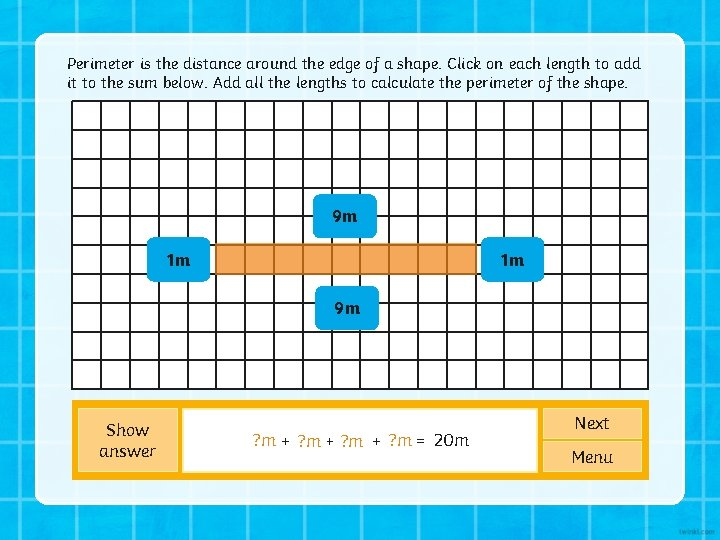Perimeter is the distance around the edge of a shape. Click on each length to add it to the sum below. Add all the lengths to calculate the perimeter of the shape. 9 m 1 m 1 m 9 m Show answer ? m = 20 m ? m + 1 m ? m + ? m 9 m 9 m + 1 m Next Menu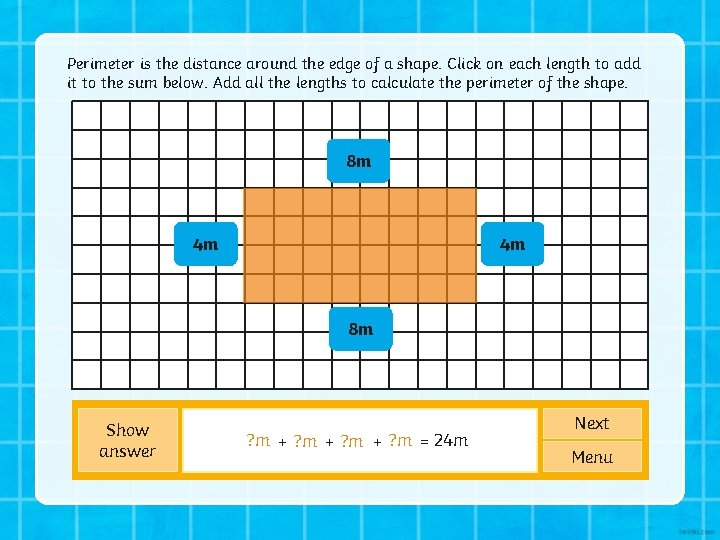Perimeter is the distance around the edge of a shape. Click on each length to add it to the sum below. Add all the lengths to calculate the perimeter of the shape. 8 m 4 m 4 m 8 m Show answer ? m = 24 m ? m + ? m 8 m 4 m + ? m 8 m + 4 m Next MenuPerimeter is the distance around the edge of a shape. Click on each length to add it to the sum below. Add all the lengths to calculate the perimeter of the shape. 6 km 2 km 6 km Show answer ? km + 2 km ? km + 6 km ? km + ? km 6 km 2 km = 16 km Next MenuPerimeter is the distance around the edge of a shape. Click on each length to add it to the sum below. Add all the lengths to calculate the perimeter of the shape. 10 cm 5 cm 10 cm Show answer ? cm + 5 cm ? cm + 10 cm ? cm + 5 cm ? cm = 30 cm Next Menu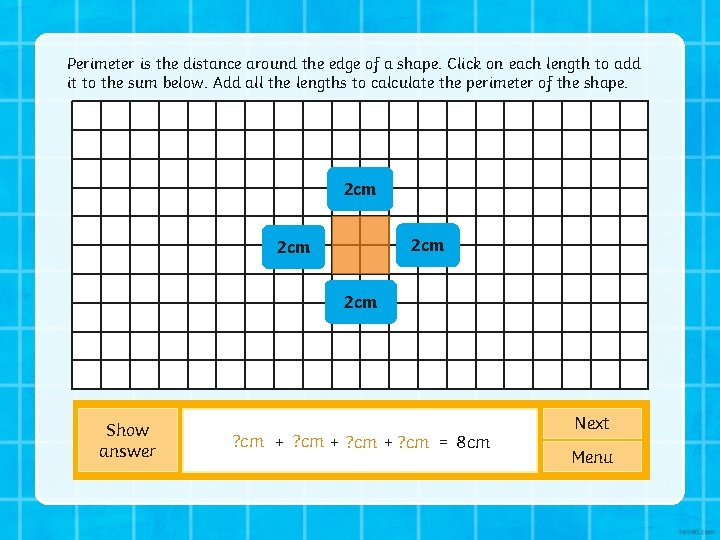Perimeter is the distance around the edge of a shape. Click on each length to add it to the sum below. Add all the lengths to calculate the perimeter of the shape. 2 cm 2 cm Show answer ? cm + 2 cm ? cm + ? cm 2 cm + 2 cm = 8 cm Next MenuPerimeter is the distance around the edge of a shape. Click on each length to add it to the sum below. Add all the lengths to calculate the perimeter of the shape. 5 cm 5 cm Show answer ? cm + 5 cm ? cm + ? cm 5 cm + 5 cm = 20 cm Next Menu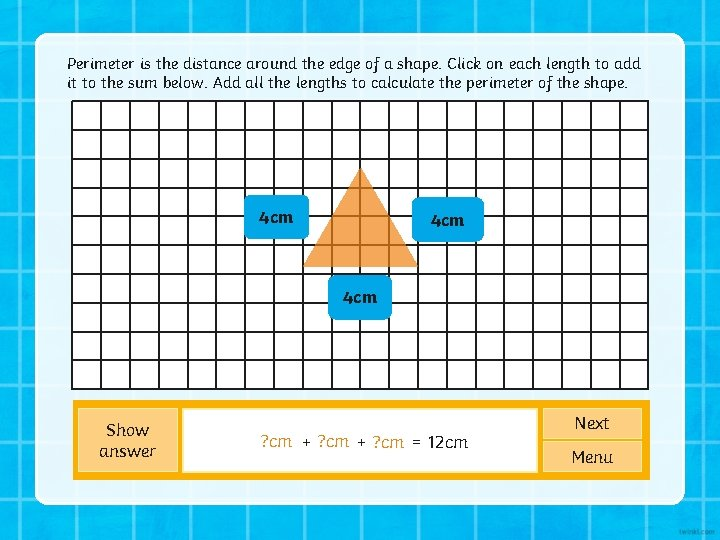Perimeter is the distance around the edge of a shape. Click on each length to add it to the sum below. Add all the lengths to calculate the perimeter of the shape. 4 cm 4 cm Show answer ? cm + ? cm 4 cm + 4 cm = 12 cm Next Menu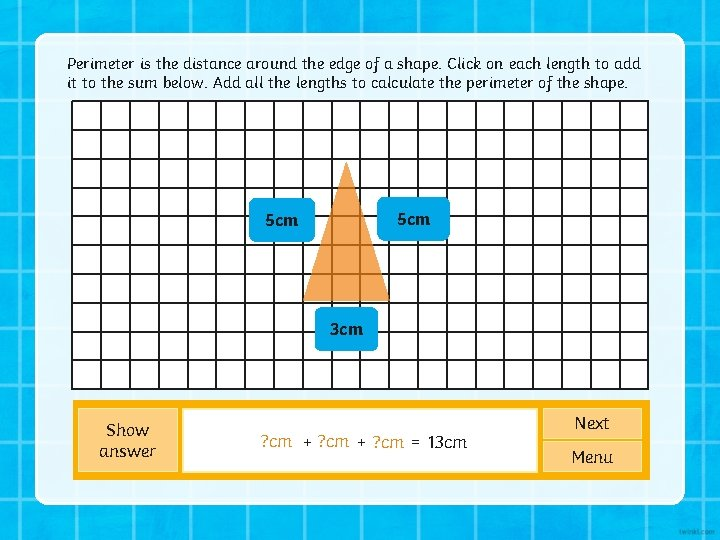Perimeter is the distance around the edge of a shape. Click on each length to add it to the sum below. Add all the lengths to calculate the perimeter of the shape. 5 cm 3 cm Show answer ? cm 5 cm + ? cm 3 cm = 13 cm Next Menu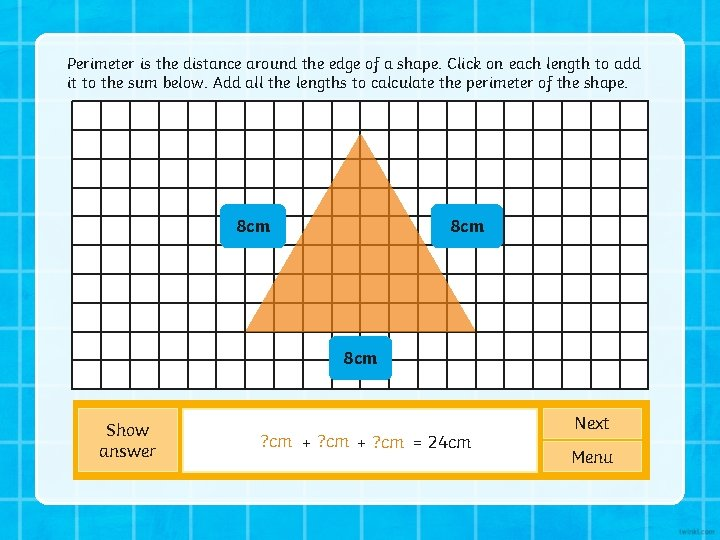Perimeter is the distance around the edge of a shape. Click on each length to add it to the sum below. Add all the lengths to calculate the perimeter of the shape. 8 cm 8 cm Show answer ? cm + ? cm 8 cm + 8 cm = 24 cm Next Menu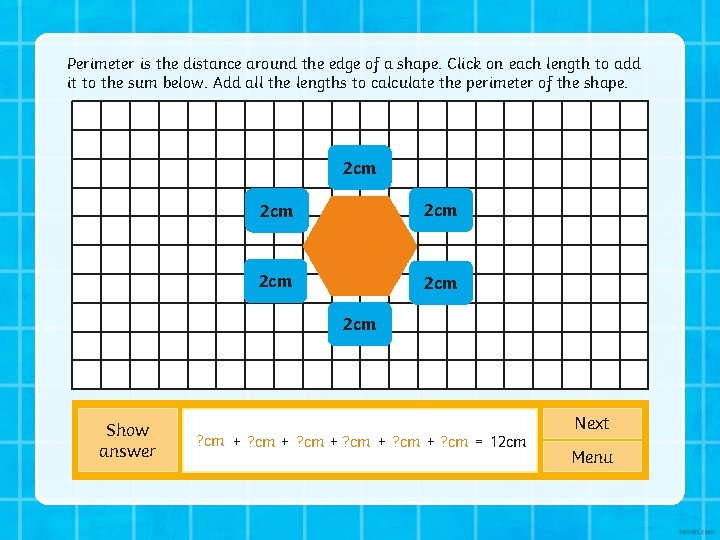Perimeter is the distance around the edge of a shape. Click on each length to add it to the sum below. Add all the lengths to calculate the perimeter of the shape. 2 cm 2 cm 2 cm Show answer ? cm + 2 cm ? cm = 12 cm ? cm + 2 cm 2 cm + 2 cm Next Menu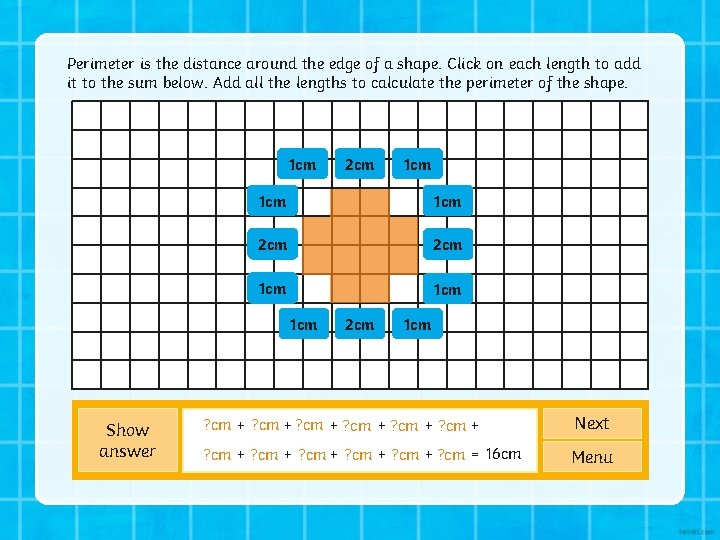Perimeter is the distance around the edge of a shape. Click on each length to add it to the sum below. Add all the lengths to calculate the perimeter of the shape. 1 cm 1 cm 2 cm 1 cm 1 cm Show answer 2 cm 1 cm ? cm + ? cm 2 cm 1 cm + ? cm 2 cm + ? cm 1 cm + Next ? cm + 2 cm ? cm + ? cm 2 cm 1 cm + ? cm 1 cm = 16 cm Menu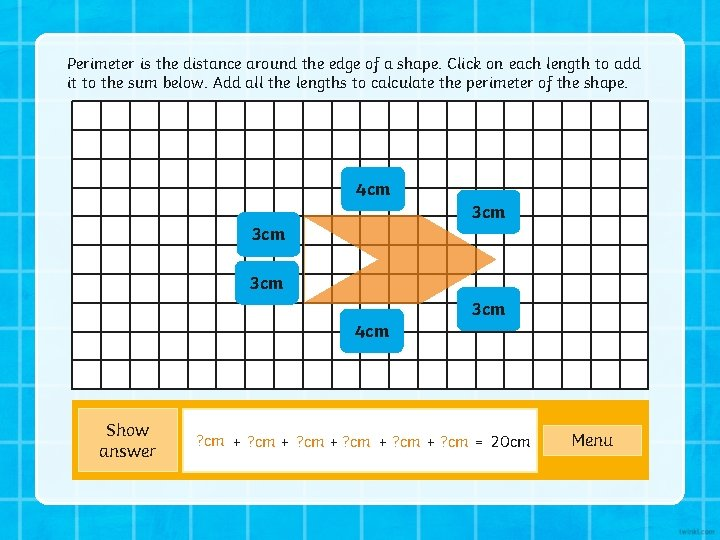Perimeter is the distance around the edge of a shape. Click on each length to add it to the sum below. Add all the lengths to calculate the perimeter of the shape. 4 cm 3 cm 3 cm 4 cm Show answer ? cm + ? cm + 3 cm 4 cm + 3 cm = 20 cm MenuPerimeter is the distance around the edge of a shape. Click on each length to add it to the sum below. Add all the lengths to calculate the perimeter of the shape. 7 cm 3 cm 7 cm Show answer ? cm + 3 cm ? cm = 20 cm 7 cm + ? cm 3 cm + 7 cm Next Menu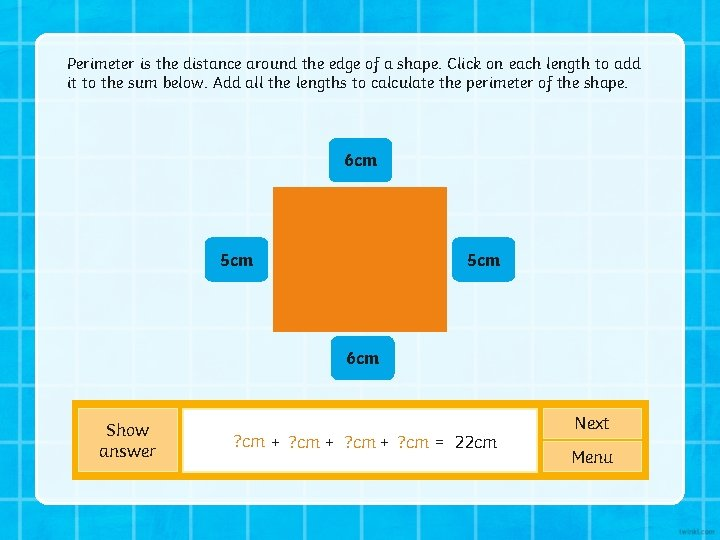Perimeter is the distance around the edge of a shape. Click on each length to add it to the sum below. Add all the lengths to calculate the perimeter of the shape. 6 cm 5 cm 6 cm Show answer ? cm + 5 cm ? cm + 6 cm ? cm + 5 cm ? cm = 22 cm 6 cm Next MenuPerimeter is the distance around the edge of a shape. Click on each length to add it to the sum below. Add all the lengths to calculate the perimeter of the shape. 9 m 1 m 1 m 9 m Show answer ? m = 20 m ? m + 1 m ? m + ? m 9 m 9 m + 1 m Next Menu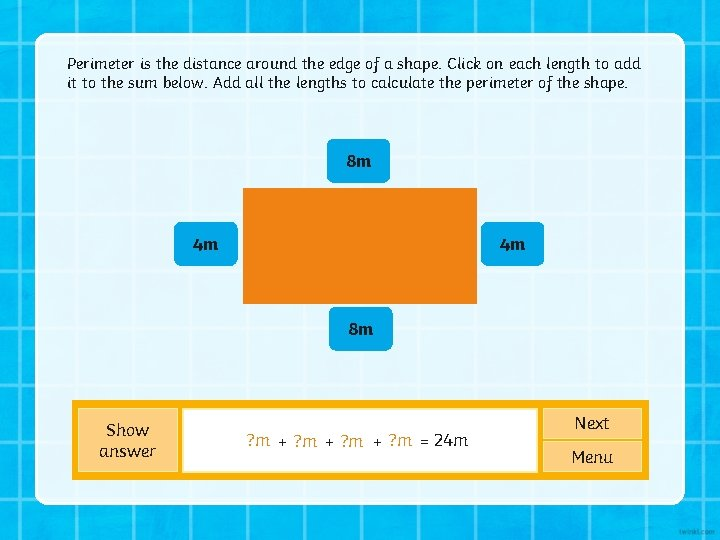Perimeter is the distance around the edge of a shape. Click on each length to add it to the sum below. Add all the lengths to calculate the perimeter of the shape. 8 m 4 m 4 m 8 m Show answer ? m = 24 m ? m + ? m 8 m 4 m + ? m 8 m + 4 m Next Menu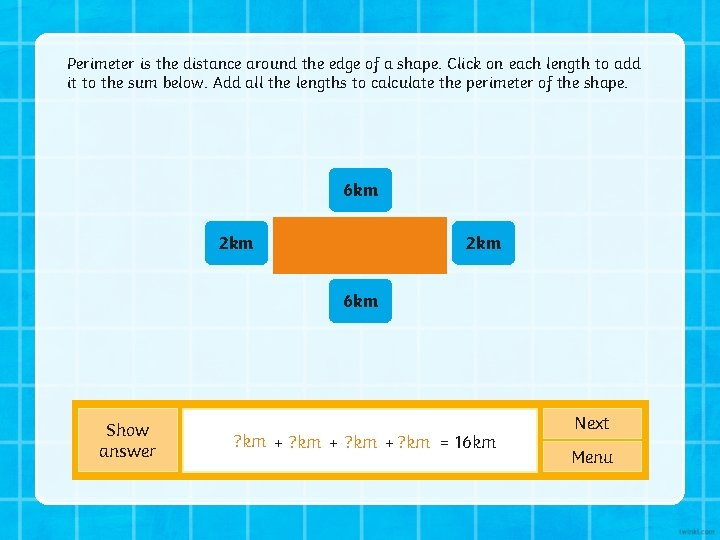Perimeter is the distance around the edge of a shape. Click on each length to add it to the sum below. Add all the lengths to calculate the perimeter of the shape. 6 km 2 km 6 km Show answer ? km 6 km + ? km 2 km + ? km 6 km + ? km 2 km = 16 km Next Menu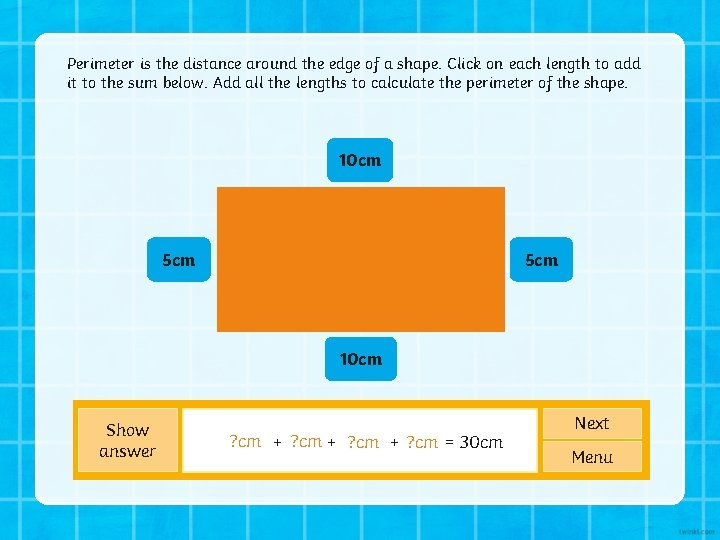Perimeter is the distance around the edge of a shape. Click on each length to add it to the sum below. Add all the lengths to calculate the perimeter of the shape. 10 cm 5 cm 10 cm Show answer ? cm + 5 cm ? cm + 10 cm ? cm + 5 cm ? cm = 30 cm Next Menu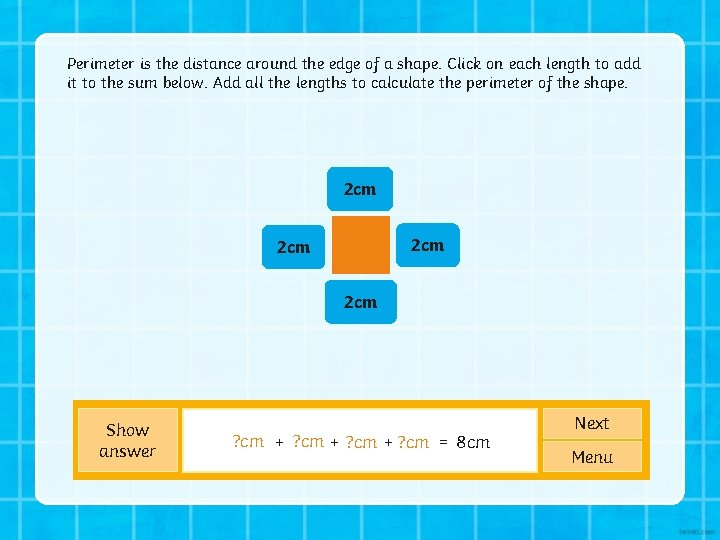Perimeter is the distance around the edge of a shape. Click on each length to add it to the sum below. Add all the lengths to calculate the perimeter of the shape. 2 cm 2 cm Show answer ? cm + 2 cm ? cm + ? cm 2 cm + 2 cm = 8 cm Next Menu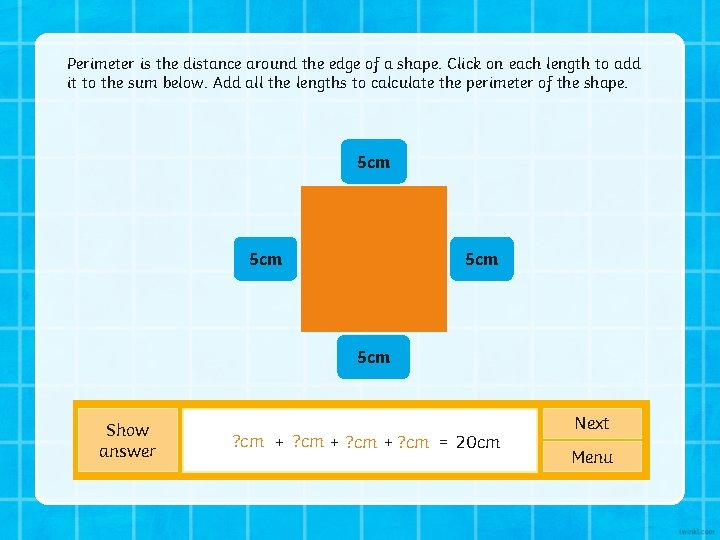Perimeter is the distance around the edge of a shape. Click on each length to add it to the sum below. Add all the lengths to calculate the perimeter of the shape. 5 cm 5 cm Show answer ? cm + 5 cm ? cm + ? cm 5 cm + 5 cm = 20 cm Next Menu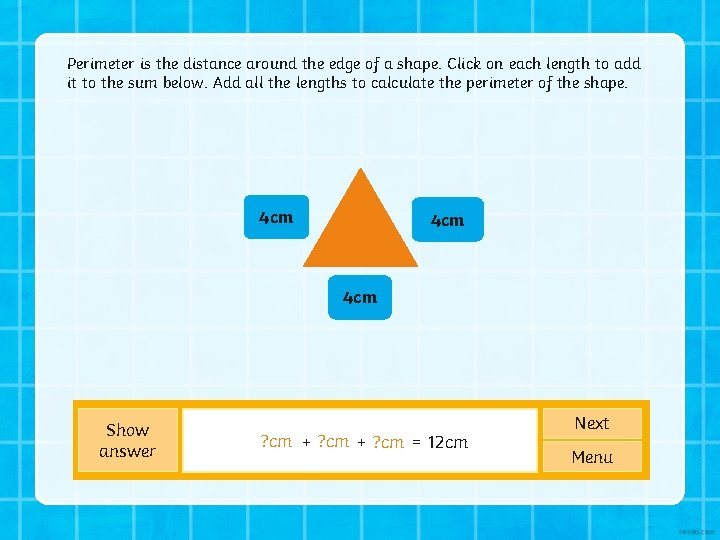Perimeter is the distance around the edge of a shape. Click on each length to add it to the sum below. Add all the lengths to calculate the perimeter of the shape. 4 cm 4 cm Show answer ? cm + ? cm 4 cm + 4 cm = 12 cm Next Menu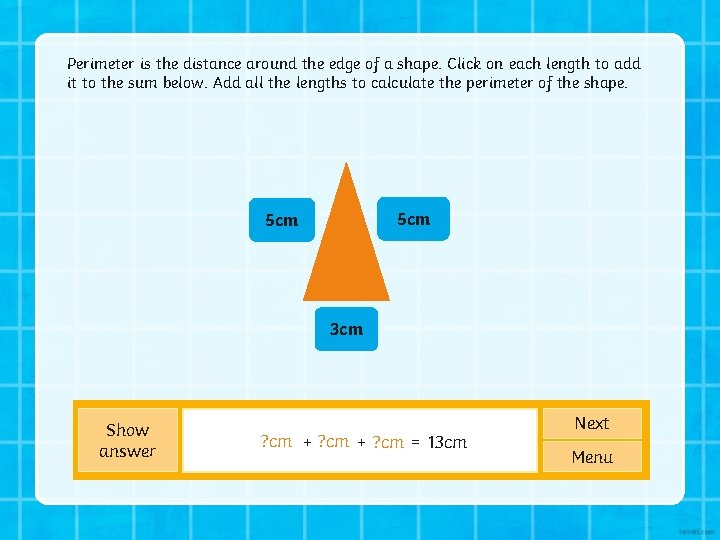Perimeter is the distance around the edge of a shape. Click on each length to add it to the sum below. Add all the lengths to calculate the perimeter of the shape. 5 cm 3 cm Show answer ? cm 5 cm + ? cm 3 cm = 13 cm Next MenuPerimeter is the distance around the edge of a shape. Click on each length to add it to the sum below. Add all the lengths to calculate the perimeter of the shape. 8 cm 8 cm Show answer ? cm + ? cm 8 cm + 8 cm = 24 cm Next Menu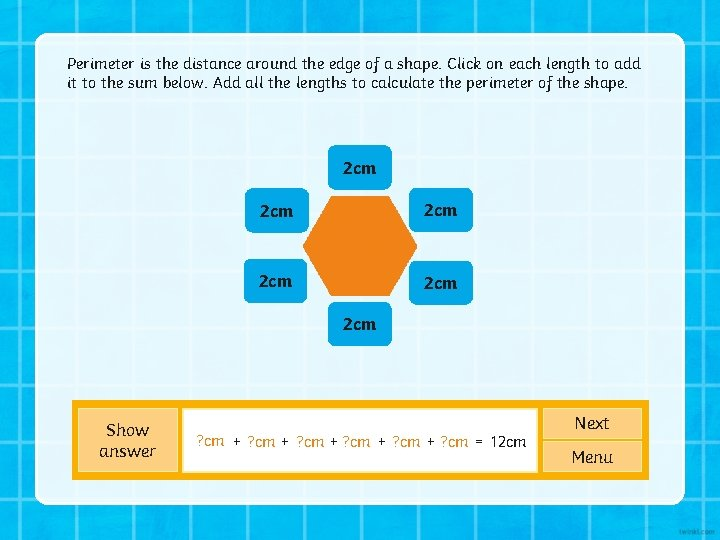Perimeter is the distance around the edge of a shape. Click on each length to add it to the sum below. Add all the lengths to calculate the perimeter of the shape. 2 cm 2 cm 2 cm Show answer ? cm + 2 cm ? cm = 12 cm ? cm + 2 cm 2 cm + 2 cm Next MenuPerimeter is the distance around the edge of a shape. Click on each length to add it to the sum below. Add all the lengths to calculate the perimeter of the shape. 1 cm 1 cm 2 cm 1 cm 1 cm Show answer 2 cm 1 cm ? cm + ? cm 2 cm 1 cm + ? cm 2 cm + ? cm 1 cm + Next ? cm + 2 cm ? cm + ? cm 2 cm 1 cm + ? cm 1 cm = 16 cm Menu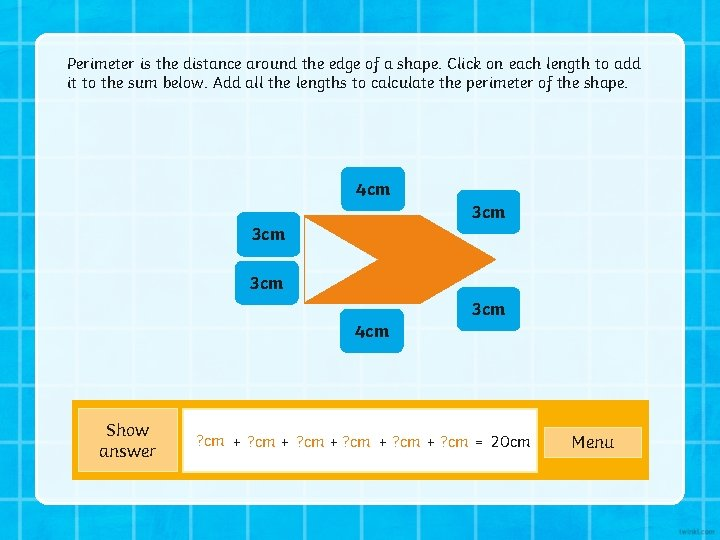Perimeter is the distance around the edge of a shape. Click on each length to add it to the sum below. Add all the lengths to calculate the perimeter of the shape. 4 cm 3 cm 3 cm 4 cm Show answer ? cm + ? cm + 3 cm 4 cm + 3 cm = 20 cm MenuNow it’s time to put your knowledge into practice. Complete the worksheet and find the perimeters of each of the shapes. Remember to complete the correct worksheet for your own group.